HOME plateWON | World!OfNumbers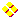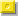Palindromic TetrahedralsFactorizationPalindromic Cubes

Introduction

Palindromic numbers are numbers which read the same from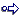left to right (forwards) as from the right to left (backwards)Here are a few random examples : 7, 3113, 44611644

Tetrahedral numbers are defined and calculated by this extraordinary intricate and excruciatingly complex formula.
So, this line is for experts only------------------------------------------ base x ( base + 1 ) x ( base + 2 )
The best way to get a 'structural' insight as how to imagine tetrahedrals is to visit these sites :

Normal and Palindromic TetrahedralsThe only numbers which are Square and Tetrahedral are 1, 4 and 19600 (Sloane's A003556)
Tetrahedral Number as proved by Meyl (1878; cited in Dickson 1952, p.25).On sci.math there was a discussion a while ago about a nonpalindromic relationship :

Question : What numbers are both Triangular and Tetrahedral ?
Answer : The only possible ones are 0, 1, 10, 120, 1540 and 7140 (Sloane's A027568).
Triangular basenumbers are 0, 1, 4, 15, 55 and 119
Tetrahedral basenumbers are 0, 1, 3, 8, 20 and 34
Proof : E.T. Avanesov (Rumanian?) proved in 1966 that the above numbers constitute the complete set of solutions.
Acta Arithmetica, vol. 12, 409-420. The proof is particularly abstruse.All tetrahedral numbers can only end with 1, 4, 5, 6 or 9. Confert the square numbers !The product of three consecutive integers is always divisible by 6.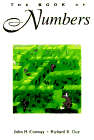A good source for such statements is "The Book of Numbers"
by John H. Conway and Richard K. Guy.
Click on the image on the left for more background about the book.
The book can be ordered at 'www.amazon.com'.The sums of the consecutive triangular numbers (starting from 1) are the tetrahedral numbers.
For example : 969 = 1 + 3 + 6 + 10 + 15 + 21 + 28 + 36 + 45 + 55 + 66 + 78 + 91 + 105 + 120 + 136 + 153
or the seventeenth tetrahedral number (17 sums).The sum of two consecutive tetrahedral numbers is a square pyramidal number.
Tn = 35 and Tn+1 = 56
Then we have 35 + 56 = 91 which is 12 + 22 + 32 + 42 + 52 + 62.The basenumber 336 leads us not only to a palindromic tetrahedral (still the record number despite its small size!)
but also to primes in one of the following manners :
336 = 6 x 7 x 8 a product of three consecutives
336 + 1 = 337 is prime.
3362 + 3372 + 3382 = 340709 is prime.The basenumber 336 is a number of the form n(n+X):
336 = n(n+5) whereby n = 16.Other interesting entries from Sloane's table are :
%N Tetrahedral numbers which are sum of 2 tetrahedrals. under A002311
%N Not the sum of 4 tetrahedrals (a finite sequence). under A000797

Sources Revealed

 Neil Sloane's "Integer Sequences" Encyclopedia can be consulted online : Neil Sloane's Integer Sequences The regular tetrahedral numbers are categorised : %N Tetrahedral numbers: C(n+3,3). under A000292. The palindromic tetrahedrals are categorised : %N nth tetrahedral number n(n+1)(n+2)/6 is palindromic under A006029. %N Palindromic tetrahedral numbers under A006030. Click here to view some of the author's [P. De Geest] entries to the table. Click here to view some entries to the table about palindromes.

The Full Listing

[ June 10, 2021 ].
Patrick De Geest's search program exhaustively scanned all possible palindromic candidates up to length 41.

[ June 1, 2002 ]
Walter Schneider (email) made a quick search up to basenumber 10^10.
No new palindromic solution was found.

Index NrInfo BasenumberLength
Palindromic TetrahedralsLength

Formula (n)(n+1)(n+2)/6
6 Info 3363
6.378.7367
5 Info 212
1.7714
4 Info Prime!    172
9693
3 Info Prime!    21
41
2 Info 11
11
1 Info 01
01

ContributionsOn General Palindromic Numbers```

```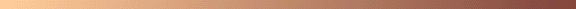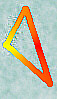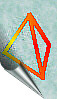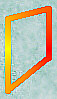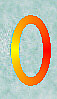[TOP OF PAGE]

Patrick De Geest - Belgium- Short Bio - Some Pictures